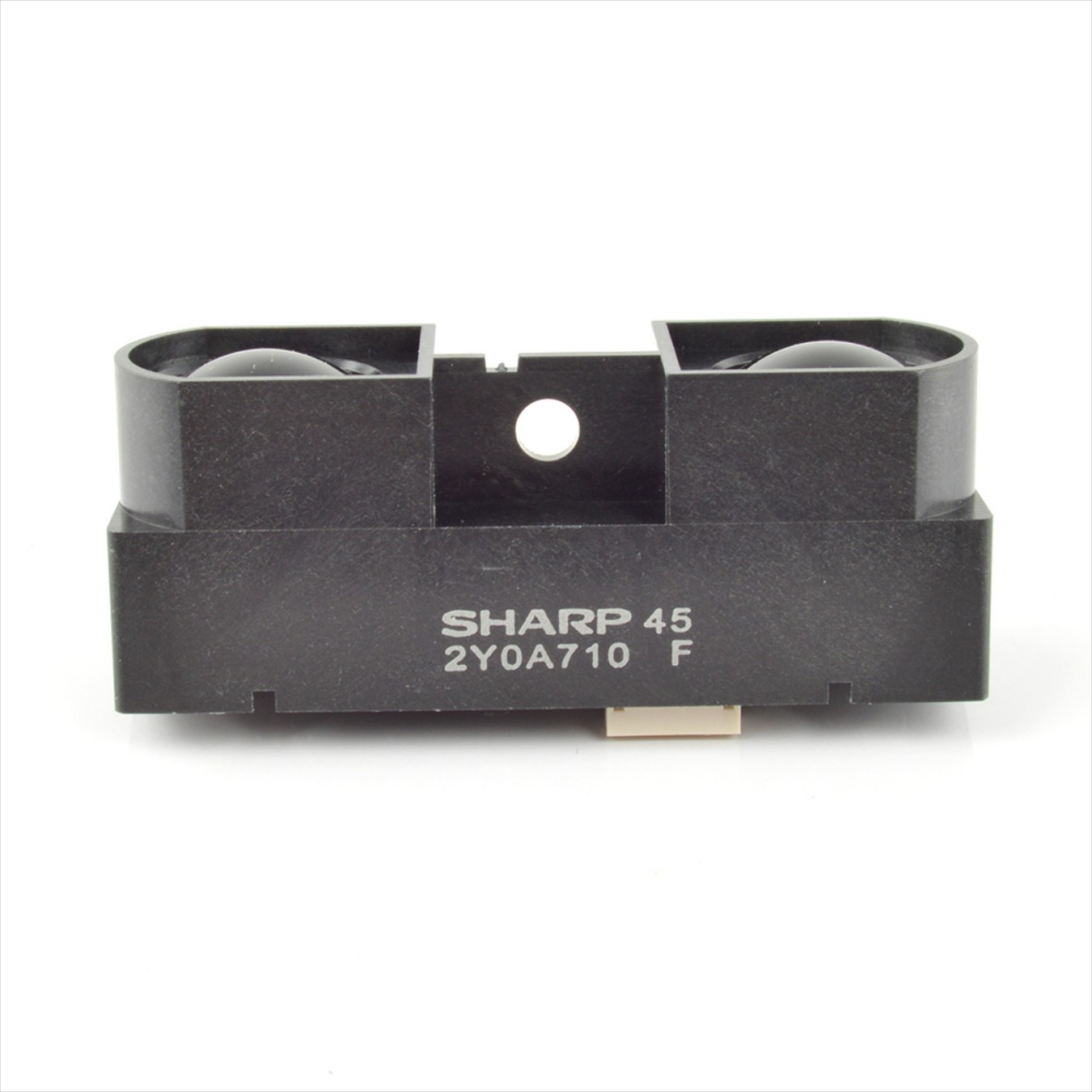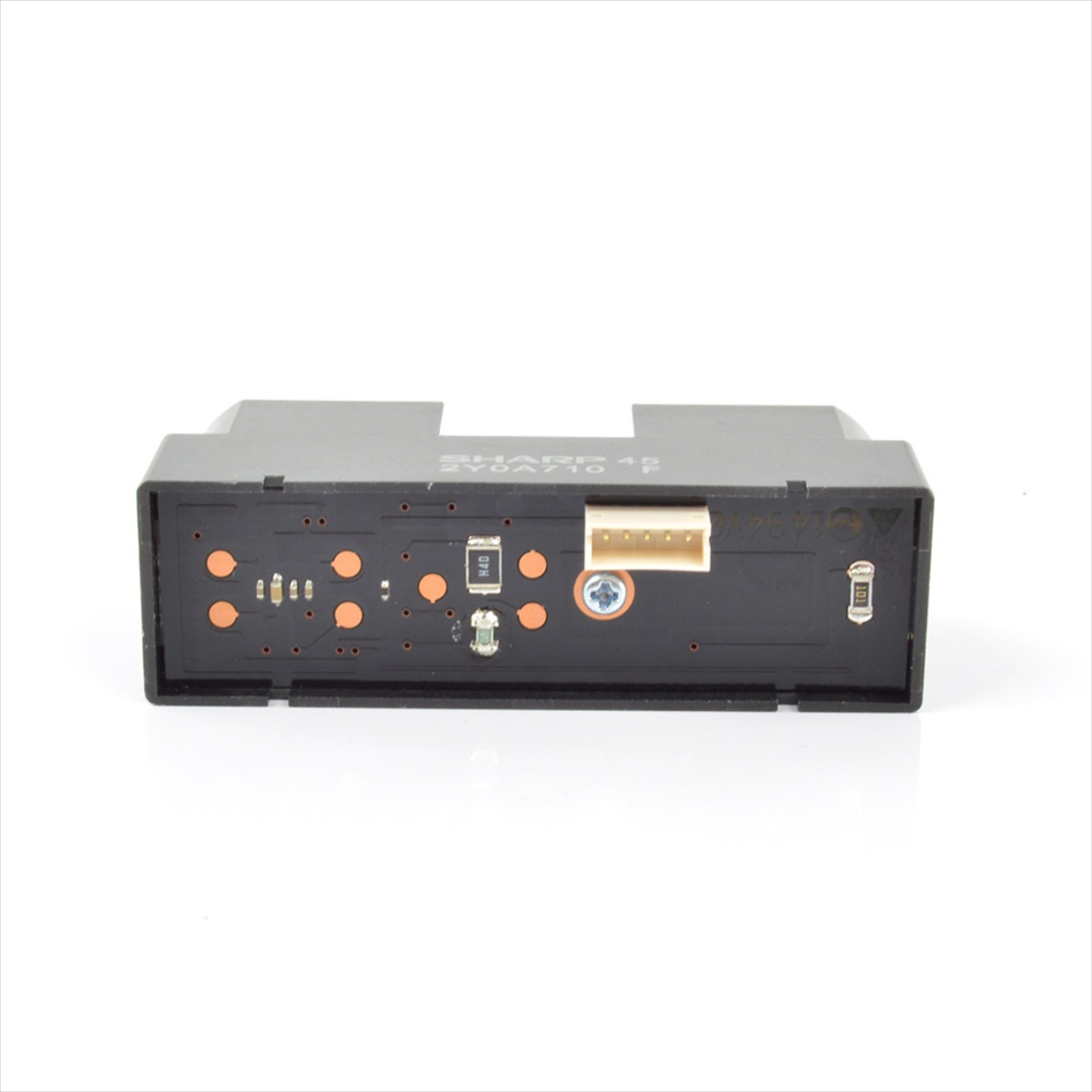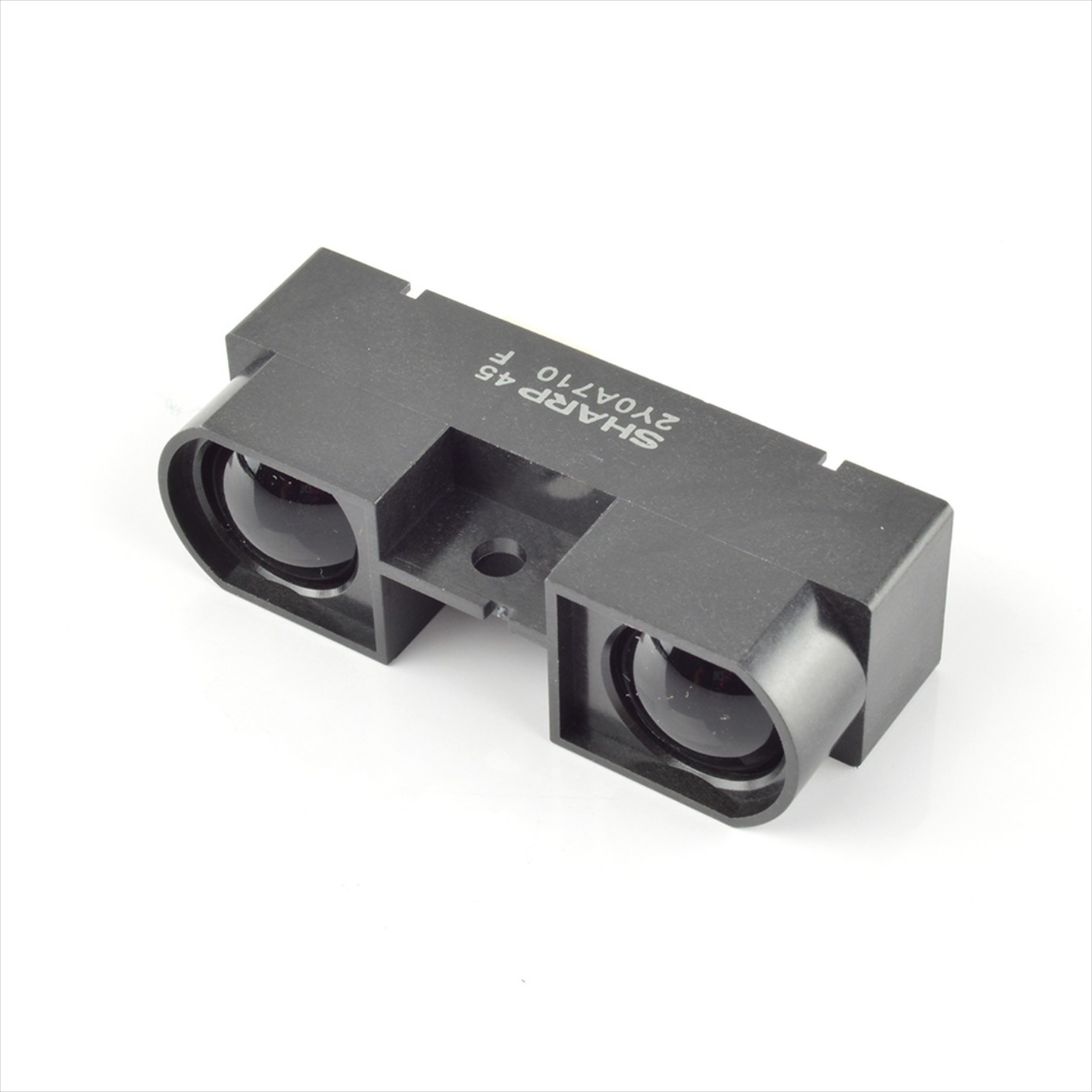# Cảm biến hồng ngoại SHARP GP2Y0A710K0F (100cm-500cm)

Thương hiệu: Chưa cập nhật |

Đánh giá

:

Giá

:

400.000₫

##### Mô tả :
Đang cập nhật ...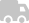Giao hàng trong và ngoài nước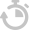Ship COD toàn quốc chỉ 22k (trọng lượng <= 2kg)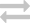Đổi trả sản phẩm trong vòng 7 ngày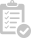Giá sỉ cho đơn hàng từ 10 sản phẩm

The GP2Y0A710K infrared sensor is a new version of GP2Y0A700K which takes a continuous distance reading and reports the distance as an analog voltage with a distance range of 1m (39.3") to 5.5m (216.5").

The interface is 5-wire JST connector (included with pins) with dual Vcc, dual Gnd, and a single output voltage.

Simply provide power and ground, and read a continuous analog voltage representing distance. A software lookup table can be implemented to translate the voltages to distances.

Two "voltage vs distance" diagrams are enough for using it. Voltage is linear to the inverse of distance. (Distance range is 1m to 5.5m)
Two reference points:

 Analog Voltage(V) Digital value(0-1023) Distance(cm) 2.5 512 100 1.4 286 500

Then, we have a linear equation of digital value and distance.
(512-sensorValue)/(1/100-1/distance)=(512-286)/(0.01-0.002)
=> distance=28250/(sensorValue-229.5)
/*Description: sharp 2Y0A710K distance ranger.(Range from 1m to 5.5m.) This code prints out data of valid range. However, if we put ranger very close to objects, we will have completely wrong results. The error is inevitable because of electric character of this device. So the only way of correct use is to make sure the distance of objects not close to the ranger. (1m to 5.5m.) Another tip: it is not very precise. So it is fit for detection,but not for measurement. Tested by Michael from DFrobot 2012/2/13*/ /*Principle of this ranger: (See details in datasheet.) Voltage is linear to the inverse of distance. (Distance range is 1m to 5.5m) Two reference points: Analog Voltage(V) Digital value(0-1023) Distance(cm) 2.5 512 100 1.4 286 500 Then, we have a linear equation of digital value and distance. (512-sensorValue)/(1/100-1/distance)=(512-286)/(0.01-0.002) => distance=28250/(sensorValue-229.5); */ void setup() { Serial.begin(9600); } int sensorValue; int distance; void loop() { sensorValue = analogRead(0); if(sensorValue>=280&&sensorValue<=512) //Corresponding distance range from 1m to 5.5m { Serial.print("The distance is: "); distance=28250/(sensorValue-229.5); Serial.print(distance); Serial.println("cm"); } delay(200); }